# Integration of Two-Dimensional Kernel Principal Component Analysis Plus Two-Dimensional Linear Discriminant Analysis with Convolutional Neural Network for Finger Vein Recognition

Integration of Two-Dimensional Kernel Principal Component Analysis Plus Two-Dimensional Linear Discriminant Analysis with Convolutional Neural Network for Finger Vein Recognition

Zhitao Gao Jianxian Cai Yanan Shi* Li Hong Fenfen Yan Mengying Zhang

Institute of Disaster Prevention, Sanhe 065201, China

Hebei Key Laboratory of Seismic Disaster Instrument and Monitoring Technology, Sanhe 065201, China

College of Electronic Science and Control Engineering, Institute of Disaster Prevention, Sanhe 065201, China

Corresponding Author Email:
caijx@cidp.edu.cn
Page:
1181-1187
|
DOI:
https://doi.org/10.18280/ts.380429
2 April 2021
|
Accepted:
5 July 2021
|
Published:
31 August 2021
| Citation

OPEN ACCESS

Abstract:

High complexity and low recognition rate are two common problems with the current finger vein recognition methods. To solve these problems, this paper integrates two-dimensional kernel principal component analysis (K2DPCA) plus two-dimensional linear discriminant analysis (2DLDA) (K2DPCA+2DLDA) into convolutional neural network (CNN) to recognize finger veins. Considering the row and column correlations of the finger vein image matrix and the classes of finger vein images, the authors adopted K2DPCA and 2DLDA separately for dimensionality reduction and extraction of nonlinear features in row and column directions, producing a dimensionally reduced compressed image without row or column correlation. Taking the dimensionally reduced compressed image as the input, the CNN was introduced to learn higher-level features, making finger vein recognition more accurate and robust. The public dataset of Finger Vein USM (FV-USM) Database was adopted for experimental verification. The results show that the proposed approach effectively overcome the common defects of original image feature extraction: the insufficient feature description, and the redundancy of information. When the training reached 120 epochs, the model basically realized stable convergence, with the loss approaching zero and the recognition rate reaching 97.3%. Compared with two-directional two-dimensional Fisher principal component analysis ((2D)2FPCA), our strategy, which integrates K2DPCA+2DLDA with CNN, achieved a very high recognition rate of finger vein images.

Keywords:

finger vein recognition, subspace learning, convolutional neural network (CNN)

1. Introduction

The recent boom of information technology (IT) has raised a growing concern about information security. Biological feature identification is widely recognized by users for its safety and convenience. This technology implements identification based on the intrinsic biometric features of the human body, which are not easily forgotten or stolen . Finger veins are a type of biometric features that support in vivo detection. There are many advantages of finger veins: they are stable, easily to acquire, difficult to copy, and recognizable without any contact. Hence, the recognition based on finger veins is superior to that based on other biometric features, such as human face , and fingerprints , attracting extensive attention from the academia.

Typically, the key to finger vein recognition algorithms lies in feature extraction and identification, which has been a research hotspot at home and abroad [4-20]. There are primarily three types of feature extraction and identification methods:

(1) Structure-based methods

The structure-based methods describe palm veins by extracting their linear or point features. The typical examples include scale-invariant Fourier transform (SIFT), which can extract locally invariant features of palm veins , and histogram of oriented gradients (HOG) .

(2) Subspace-based methods

Treating each palm vein image as a high-dimensional vector or matrix, subspace-based methods convert the image into a low-dimensional vector or matrix through projection or transformation, and represent and match palm veins in the low-dimensional space. The typical examples include two-directional two-dimensional linear discriminant analysis (2D)2LDA , principal component analysis (PCA) , and singular value decomposition (SVD)-based minutiae matching (SVDMN) .

(3) Texture-based methods

The texture-based methods firstly extract the direction, frequency, and phase of the local features of the palm vein texture image, treat them as palm vein features, and then encode the features for matching and recognition. The typical examples include Gabor filter , local binary pattern (LBP) , and pose part-based model .

The above methods achieve desired results from different perspectives. However, each type of methods has its defects. Structure-based methods can describe the local shapes, but the descriptor is generated slowly over a long period, i.e., the real-time performance is poor. Subspace-based methods can reduce dimensionality, but at the cost of simple computing. Texture-based methods, such as Liu et al.’s SVDMN extended from SVD , usually computes the discriminant similarity of local features around detail points like the intersections and ends of veins. Nonetheless, the extracted detail points are insufficient for feature matching, when the veins are sparse. For dimensionality reduction and extraction of the linear features of finger veins, some scholars have developed two-way two-dimensional principal component analysis (2D)2PCA algorithms, namely, two-dimensional principal component analysis (2DPCA), two-dimensional Fisher's linear discriminant (2DFLD), and two-dimensional linear discriminant analysis (2DLDA). However, these algorithms overlook the nonlinear correlations between features, failing to thoroughly extract finger vein features.

In recent years, deep learning (DL)  has made great breakthroughs in the fields of computer vision, such as image classification [22-24], image recognition, image detection , image segmentation , etc. Notably, convolutional neural network (CNN)  achieved remarkable success in many aspects, ranging from human action identification action identification , signal reconstruction [29, 30], and other applications.

Considering the research status above and the characteristics of finger vein recognition, this paper integrates two-dimensional kernel principal component analysis (K2DPCA) plus 2DLDA (K2DPCA+2DLDA) into CNN to recognize finger veins. Specifically, K2DPCA was adopted to extract nonlinear features, solving the problem of extracting nonlinear separable features of finger veins; 2DLDA was employed for supervised linear dimensionality reduction and extraction of features, aiming to reduce redundant information; CNN was introduced to learn higher-level semantic features. Experimental results show that our approach achieved a good recognition effect on a public database.

2. Finger Vein Recognition Based on K2DPCA+2DLDA and CNN

This paper combines K2DPCA+2DLDA and CNN into a novel way to recognize finger veins. Firstly, nonlinear dimensionality reduction and feature extraction were carried out with K2DPCA+2DLDA to screen out the massive redundant information in the original image. Next, the CNN was introduced to extract and learn the higher-level semantic features (Figure 1).

For an input image I of the size r×c, our method involves the following steps:

Stage 1:

Input: Finger vein region-of-interest (ROI) image $A^{m \times n}$.

Output: Finger vein feature data $C^{d \times r}$.

Step 1. Perform K2DPCA transform of the input image $A^{m \times n}$ in the row direction to obtain the projection of the mapping $\Phi(A)$ of image $\mathrm{A}$ in feature space $\mathrm{F}$ on eigenvector, producing the corresponding feature matrix $Y^{m \times r}$.

Step 2. Perform 2 DLDA transform of the feature matrix in the column direction to obtain the transform matrix $V$, and transpose $V$ into $V^{T}$.

Step 3. Reduce the dimensionality of the data again with the transposed matrix $V^{T}$, yielding the final feature $C^{d \times r}=$ $V^{T} Y^{m \times r}=V^{T} .$

Stage 2:

Import the dimensionality reduced training set obtained in the above stage into the designed CNN, and train the network through forward propagation and error backpropagation. After the training, import the training set into the network, and obtain the recognition result through forward propagation.

## 1.pngFigure 1. Principle of K2DPCA+2DLDA combined with CNN

2.1 K2DPCA

The high-dimensional computation of PCA might result in the curse of dimensionality, while 2DPCA lacks the ability to extract nonlinear features. In this paper, these two problems are overcome by K2DPCA .

As a nonlinear feature extractor, K2DPCA nonlinearly maps each row of the input image matrix to a high-dimensional space, and performs the PCA transform in that space. However, it is very difficult to solve the eigenvalues and eigenvectors of the scatter matrix corresponding to the data mapped to the high-dimensional space. To avoid complex direct calculation, this paper overcomes the difficulty by solving the eigenvalues and eigenvectors of kernel matrix. The specific steps are as follows:

Let m be the total number of training samples; Xk be the k-th training sample; Xki be the i-th row vector of the k-th training sample; X=[X1,X2,……,Xm], Xi=[(Xi1)T,(Xi2)T,……,(Xim)T]T; Ф is the corresponding nonlinear mapping.

By inner product kernel function K, the inner product of the projections of input data Xi and Xj in higher-dimensional space F can be calculated by:

$K\left(X_{i}, X_{j}\right)=\Phi\left(X_{i}\right) \cdot \Phi\left(X_{j}\right)=\Phi\left(X_{i}\right) \Phi\left(X_{j}\right)^{T}$                  (1)

The mapping $\widehat{\Phi}$ can be established through the centralization of all data. Then, the covariance matrix $C^{\Phi}$ of space $\mathrm{F}$ can be expressed as:

$C^{\Phi}=\frac{1}{m} \sum_{i=1}^{m} \widehat{\Phi}\left(X_{i}\right) \widehat{\Phi}\left(X_{j}\right)^{T}$                (2)

where, $\widehat{\Phi}\left(X_{i}\right)=\left[\widehat{\Phi}\left(X_{i}^{1}\right), \widehat{\Phi}\left(X_{i}^{2}\right), \ldots \ldots, \widehat{\Phi}\left(X_{i}^{m}\right)\right]$. By solving the eigenvalues and eigenvectors of the covariance matrix, there is no need to conduct complex computation with every column vector.

$\operatorname{Each} \widehat{\Phi}\left(X_{i}^{j}\right)$ can be projected to the eigenvector xk of space F:

$x_{k} \widehat{\Phi}\left(X_{i}^{j}\right)^{T}=\sum_{p=1}^{m} \sum_{q=1}^{n} \alpha_{l}^{p \times q}\left(\widehat{\Phi}\left(X_{p}^{q}\right) \widehat{\Phi}\left(X_{i}^{j}\right)^{T}\right)$                  (3)

$=\sum_{p=1}^{m} \sum_{q=1}^{n} \alpha_{l}^{p \times q} \widehat{K}\left(X_{p}^{q}, X_{i}^{j}\right)$            (4)

where, l=m×n-d+1, m×n-d+1,……, m×n.

Then, the projection Yi of the i-th mapping image $\widehat{\Phi}\left(X_{i}\right)$ can be obtained as:

$Y_{i}=x_{k} \widehat{\Phi}\left(X_{i}\right)=\alpha^{T}\left(\Psi^{\Phi}\right)^{T} \widehat{\Phi}\left(X_{i}\right)$                 (5)

where,

$\alpha=\left(\alpha_{m \times n-d+1}, \alpha_{m \times n-d+2}, \ldots, \alpha_{m \times n}\right)$              (6)

$\Psi^{\Phi}=\left[\left[\widehat{\Phi}\left(X_{1}^{1}\right), \ldots, \widehat{\Phi}\left(X_{1}^{n}\right)\right], \ldots,\left[\widehat{\Phi}\left(X_{m}^{1}\right), \ldots, \widehat{\Phi}\left(X_{m}^{n}\right)\right]\right]$             (7)

The row vectors of all training images are projected to the first d eigenvectors in the feature space. Then, the features in the row direction can be extracted from the projection matrix of each image obtained through K2DPCA transform.

2.2 2DLDA

To extract features in the column direction, this paper chooses 2DLDA proposed by Ming Li et al., which follows similar ideas as 2DPCA. Like 2DPCA, 2DLDA directly reduces the dimensionality on the image matrix, and thus avoids the heavy load of vector computation, without sacrificing the dimensionality reduction performance. The steps of 2DLDA are as follows:

Suppose the training set contains M images in c classes. Let Ai,j be an m×n matrix of the j-th image in class i. Let $\bar{A}$ be the mean matrix of all training samples; $\bar{A}_{i}$ be the mean of class i images. Then, the inter-class scatter matrix $S_{b}^{r o w}$ and the intra-class scatter matrix $S_{w}^{\text {row }}$ can be respectively written as:

$S_{b}^{\text {row }}=\frac{1}{M} \sum_{i=1}^{C}\left(\overline{A_{i}}-\bar{A}\right)^{T}\left(\overline{A_{i}}-\bar{A}\right)$          (8)

$S_{w}^{r o w}=\frac{1}{M} \sum_{i=1}^{C} \sum_{j=1}^{n}\left(A_{i, j}-\bar{A}_{i}\right)^{T}\left(A_{i, j}-\bar{A}_{i}\right)$              (9)

Sorting the eigenvalues of $\left(S_{w}^{r o w}\right)^{-1} S_{b}^{r o w}$ in descending order, the eigenvectors corresponding to the top-q eigenvalues can be combined into a mapping axis:

$V=\left(v_{1}, v_{2}, \ldots, v_{q}\right)$                   (10)

Finally, the matrix is mapped to axis V to obtain the eigenmatrix of each training image, and maximize the total scatter matrix. After 2DLDA dimensionality reduction, the eigenmatrix C of Y can be obtained as:

$C=V^{T} Y$                  (11)

K2DPCA and 2DLDA have a common defect: they are only capable of eliminating the correlation in one direction via feature compression. The features can only be extracted in the row or column direction in the 2D image. It is impossible to eliminate the correlations in both column and row directions. To overcome the limitation, this paper couples K2DPCA with 2DLDA. Firstly, K2DPCA transform was applied to the image matrix in the row direction to eliminate row correlation; Next, 2DLDA transform was implemented in the column direction to eliminate column correlation. In this way, a dimensionally reduced compressed image could be obtained without row or column correlation. Figure 2 shows the process of K2DPCA+2DLDA method. In addition, supervised learning was introduced to classify the information, which simplifies the computation, improves recognition accuracy, increases compression rate, and saves storage space. As a result, the proposed algorithm K2DPCA+2DLDA is expected to have a good performance.

## 2.pngFigure 2. Process of K2DPCA+2DLDA transform

2.3 DL

DL, an extension of traditional machine learning, has been widely applied in many fields, because it can automatically learn the suitable representation of features. During image recognition, general DL algorithms would lose the structural information of the original image, exerting a negative impact on recognition effect. As a DL network, CNN performs convolution with the aid of local receptive fields, which preserves the structural relationship of the original signal space. Besides, weights are shared to reduce the parameters to be trained. Hence, the CNN has been extensively implemented in image recognition.

CNN is a deep feed-forward neural network, whose structure is easy to set up and train. Compared with the earlier fully-connected neural network, the CNN boasts excellent generalization and robustness. The efficient network architecture attracts wide attention from computer vision teams. The classic structure of the CNN encompasses convolutional layers and a fully-connected layer. Normally, a convolutional layer involves convolution, nonlinear activation, and pooling operations. During the learning, the training set is inputted to the convolutional layers in the front of the network for learning and training, while the overall feature is integrated in the fully-connected layer in the rear. On this basis, more abstract higher-level semantics are learned through training. The defining feature of CNN structure is the adoption of three key processes: local receptive field processing, weight sharing mechanism, and pooling technology. These processes not only reduce the computing load, but also enhance network robustness and generalization.

(1) Convolutional layer

Different features are extracted from the input image via the local receptive fields, using convolution kernels. The kernels of any dimension need to completely traverse the input, and share weights and biases through the weight sharing mechanism. In this way, the kernels of any dimension can extract the corresponding abstract information. Drawing on the principle of local image perception, the computation can be speed up by defining different strides for local receptive fields, and the bias sharing can reduce the target parameters in the network. In this paper, the kernel size 1×1 is applied before the kernel size 3×3 (Figure 3). Instead of changing height or width, the 1×1 kernel increases or reduces the original data volume by changing the number of channels. Under the premise of scale invariance, the kernel can substantially enhance nonlinear features, and deepen the network, thereby improving the expression ability and increasing the number of parameters. Meanwhile, 3×3 kernels promote information exchange between channels, making finger vein recognition more accurate.

## 3.png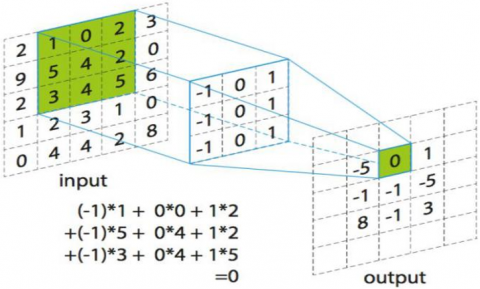Figure 3. Convolution operation

(2) Nonlinear layer

The nonlinear layer generally receives the results from the previous layer, and then performs nonlinearization on the results. This layer introduces more nonlinearity to the model, and enables the model to better fit nonlinear targets, making up for the defect of linear models. There are many commonly used nonlinear activation functions, including sigmoid, rectified linear unit (ReLU), and Leaky ReLU. This paper chooses ReLU as the activation function. Unlike sigmoid, ReLU has a relatively small computing load, and rarely encounter vanishing gradients. It is a desirable tool for deep network training. ReLU makes some nodes to output zero, which ensures network sparsity, reduces parameter interdependence, and mitigates overfitting (Figure 4).

## 4.png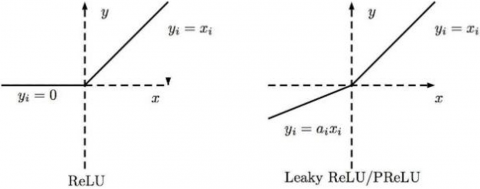Figure 4. Nonlinear activation function

(3) Pooling layer

The pooling layer essentially downs samples the output of the previous layer, aiming to preserve feature description ability while reducing image resolution. This layer often comes right after a convolutional layer to simplify the convolutional output. There are two types of operations of the pooling layer: average pooling and max pooling. Finger vein recognition mainly targets images, which only contain a few useful information in the convolution process. Most information of images is redundant. Max pooling (Figure 5) helps to prevent the interference of lots of redundant information. In addition, average pooling can reduce the first type of error (increase of estimation variance caused by the limited neighborhood size), and preserve the most of background information; max pooling can reduce the second type of error (shift of mean estimation induced by the error of convolutional parameters), and preserve texture information. This paper adopts max pooling, because finger vein recognition needs to preserve much of the texture information.

As shown in Table 1, our CNN has four learning layers, including 2 convolutional (conv) layers and 2 fully-connected (fc) layers. During the training, the probability of overfitting was reduced through dropout regularization, the training speed was increased with ReLU, and the recognition accuracy was enhanced through pooling and normalization.

## 5.png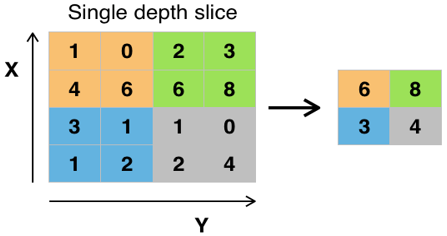Figure 5. Max pooling with stride of 1 and operator of 2×2

Table 1. CNN parameters

 Layer Layer Type Size 1 Convolution 64, 1×1, stride:2 1 ReLU － 1 Max Pooling 3×3, stride:1 1 Dropout p:0.2 2 Convolution 64, 3×3, stride:1 2 ReLU － 2 Max Pooling 3×3, stride:1 2 Dropout p:0.5 3 Full connection 2048 3 ReLU － 4 Full connection 512

The original finger vein image has several defects, namely, redundant information, high-dimensional data, and lack of information representation. These defects can be effectively solved by the proposed K2DPCA+2DLDA, coupled with CNN.

3. Experiments and Results Analysis

3.1 Experiments on K2DPCA+2DLDA+CNN

Our experiments were carried out on Finger Vein USM (FV-USM) Database, which was proposed by Prof. Bakhtiar Affendi Rosdi at Universiti Teknologi Malaysia (UTM). The database offers images on the left index finger, left middle finger, right index finger, and right middle finger of 83 males and 40 females. Six images were taken on each of the four fingers of every subject. The spatial and depth resolutions of the images are 640×480 and 256 (grayscale). In total, 123×4×6=2,952 images were acquired in 492 classes.

## 6.pngFigure 6. Finger vein feature extraction

As shown in Figure 6, the original dataset was expanded by rotating each image by 0°, ±1°, and ±2°, turning the original 2,952 samples to 13,260 samples. Then, the ROI was extracted from each image, and subjected to scale normalization.

Cross-validation was adopted for our experiments. Out of the 30 same-class samples of each finger, 25 were randomly selected to form a training set, and the remaining 5 were grouped into a test set. Hence, our training set has a total of 11,050 samples, and our test set has a total of 2,210 samples. The training was accelerated by mini-batch strategy and graphics processing unit (GPU). Under Adam optimizer, the batch size was set to 500, the training time to 120 epochs, and the learning rate to η=0.0001. L2 regularization was adopted with the parameter λ=0.0005.

## 7.png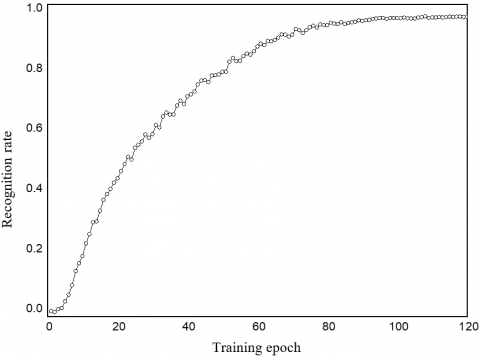Figure 7. Training recognition rate of K2DPCA+2DLDA+CNN

## 8.png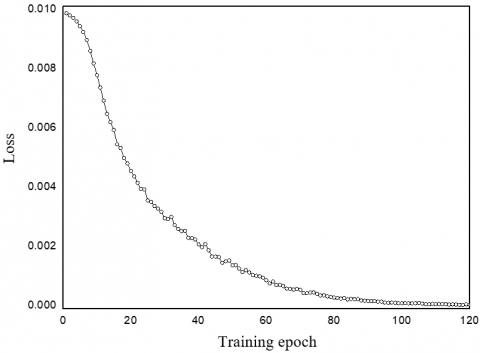Figure 8. Training loss of K2DPCA+2DLDA+CNN

Figures 7 and 8 show the variation of recognition rate and loss with the iterative training epochs. Under the iteration of Adam optimizer + L2 regularization, the greater the training epochs, the higher the recognition rate, and the lower the training loss. When the training reached 120 epochs, the model basically realized stable convergence, with the recognition rate reaching 97.3%.

3.2 Comparison with (2D)2FPCA

To verify its recognition effect, the proposed K2DPCA+2DLDA+CNN was compared with the (2D)2FPCA, which is improved from classic pattern recognition algorithms like PCA and FLD. Both recognition methods were applied on the FV-USM dataset. The recognition rate of each method was calculated. The experimental results of (2D)2FPCA are recorded in Table 2.

Table 2. Recognition rates of (2D)2FPCA under different feature dimensions

 Feature dimension Recognition rate % False accept rate (FAR) % 6×3 95.83 4.17 10×5 94.12 5.88

Under the dimensionality of 6×3, the training process (Figure 9) and feature mapping (Figure 10) of the ROI image on finger veins were obtained. Our method firstly derives the mean map (Figure 9(a)) of all finger veins in the training set, performs 2DPCA transform on the mean map to obtain Figure 9(b), and then performs 2DFLD transform to obtain Figure 9(c). Obviously, the extracted features are already very abstract. Meanwhile, the ROI image (Figure 10(a)) was directly imported to (2D)2FPCA, which outputted a feature map (Figure 10(b)) through transform and mapping. The feature map contains highly abstracted features.

## 9aaa.jpg(a) Mean map of training set

## 9bbb.jpg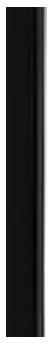(b) Result of 2DPCA transform on mean map

## 9ccc.jpg(c) Result of 2DFLD transform

Figure 9. Training process

## 10.jpg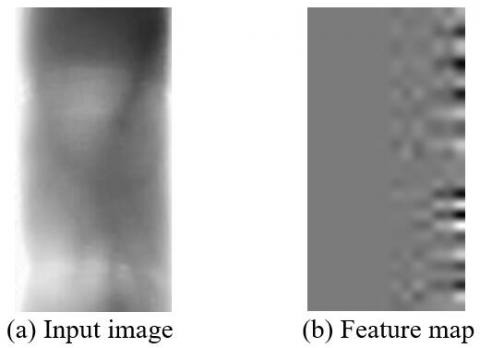Figure 10. Before and after feature mapping

Further, the proposed K2DPCA+2DLDA+CNN was compared with (2D)2FPCA, and SIFT in terms of recognition effect. The comparison results are shown in Table 3.

Table 3. Comparison of recognition effects

 Method Recognition rate % FAR % K2DPCA+2D LDA+CNN 97.3% 2.7% (2D)2FPCA 95.83% 4.17% SIFT 96.6% 6.3%

The above experiments demonstrate that the proposed K2DPCA+2DLDA+CNN is highly accurate, and works well on the classification of finger vein images.

4. Conclusions

Considering the characteristics of finger veins, this paper integrates K2DPCA+2DLDA with CNN to process finger vein images, and verifies the proposed approach through experiments on FV-USM dataset. The following conclusions were draw:

(1) Concerning the finger vein image, the K2DPCA+2DLDA and supervised learning can reduce dimensionality through training more accurately, extract nonlinear features more effectively, compress the image greatly, and remove redundant information from the image. In this way, the data volume is reduced, the data representation is improved, and the data are depicted accurately.

(2) The CNN was introduced to train and learn the finger vein images after dimensionality reduction, and to extract higher-level features. After that, the recognition rate could reach as high as 97.3%.

(3) The proposed K2DPCA+2DLDA+CNN is much more accurate in recognizing finger vein images than (2D)2FPCA.

Acknowledgments

This work was supported by the Fundamental Research Funds for the Central Universities (Grant No.: ZY20180111), the Fundamental Research Funds for the Central Universities (Grant No.: ZY20180112), the Fundamental Research Funds for the Central Universities (Grant No.: ZY20210318), and the Fundamental Research Funds for the Central Universities (Grant No.: ZY20210319).

References

 Unar, J,A., Seng, W,C., Abbasi, A. (2014). A review of biometric technology along with trends and prospects. Pattern Recognition, 47(8): 3673-2688. https://doi.org/10.1016/j.patcog.2014.01.016

 Xu, L.F., Li, Y.Q., Hu, M., Liu, S.Z., Wang, X.H. (2015). Face recognition method based on I-DCV. Journal of Electronic Measurement and Instrumentation, 29(1): 106-110. http://dx.chinadoi.cn/10.13382/j.jemi.2015.01.014

 Bi, X.Q., Su, Y.J., Wang, Q. (2015). Design and experimental study of embedded fingerprint  identification system. Foreign Electronic Measurement Technology, 34(2) : 50-53.

 Prommegger, B., Uhl, A. (2021). A fully rotation invariant multi‐camera finger vein recognition system. IET Biometrics, 10(3): 275-289. https://doi.org/10.1049/bme2.12019

 Titrek, F., Baykan, Ö.K. (2020). Finger vein recognition by combining anisotropic diffusion and a new feature extraction method. Traitement du Signal, 37(3): 433-441.https://doi.org/10.18280/ts.370310

 Ren, H., Sun, L., Guo, J., Han, C., Wu, F. (2021). Finger vein recognition system with template protection based on convolutional neural network. Knowledge-Based Systems, 227: 107159. https://doi.org/10.1016/j.knosys.2021.107159

 Liu, H., Yang, G., Yin, Y. (2020). Category-preserving binary feature learning and binary codebook learning for finger vein recognition. International Journal of Machine Learning and Cybernetics, 11(11): 2573-2586. https://doi.org/10.1007/s13042-020-01143-1

 Ganugula, S.H., Rayudu, S., Bharadwaj, K.A., Saravanan, K. (2020). Finger Vein recognition using Vgg-16 CNN algorithm. International Journal of Innovative Technology and Exploring Engineering (IJITEE), 9(8): 112-114. https://doi.org/10.35940/ijitee.H6254.069820

 Luo, R., Zhang, K. (2020). Research on finger vein recognition based on improved convolutional neural network. International Journal of Social Science and Education Research, 3(4): 107-114. http://doi.org/10.6918/IJOSSER.202004_3(4).0014

 Bao, X.A., Yi, R., Xu, L.,Wu, B., Zhong, L.H. (2020). Improved residual network for finger vein recognition. Journal of Xi'an Polytechnic University, 34(3): 67-74. http://dx.chinadoi.cn/10.13338/j.issn.1674-649x.2020.03.011

 Xie, C., Kumar, A. (2019). Finger vein identification using convolutional neural network and supervised discrete hashing. Pattern Recognition Letters, 119: 148-156.https://doi.org/10.1016/j.patrec.2017.12.001

 Kang, W., Liu, Y., Wu, Q., Yue, X. (2014). Contact-free palm-vein recognition based on local invariant features. PloS One, 9(5): e97548. https://doi.org/10.1371/journal.pone.0097548

 Computation - Neural Computation; New Findings in Neural Computation Described from University of Shandong (Learning Personalized Binary Codes for Finger Vein Recognition). (2019). Journal of Robotics & Machine Learning.

 Xu, X., Yao, P. (2016). Palm vein recognition algorithm based on HOG and improved SVM. Computer Engineering and Applications, 11: 175-180.

 Lee, Y.P. (2015). Palm vein recognition based on a modified [formula]. Signal, Image and Video Processing, 9(1): 229-242. https://doi.org/10.1007/s11760-013-0425-6

 Li, Q. (2010). Theoretical and experimental research on palm vein identification technology. Wuhan: Huazhong University of Science and Technology.

 Liu, F., Yang, G., Yin, Y., et al. (2014). Singular value decomposition based minutiae matching method for finger vein recognition. Neurocomputing, 14: 75-89. https://doi.org/10.1016/j.neucom.2014.05.069

 Mirmohamadsadeghi, L., Drygajlo, A. (2014). Palm vein recognition with local texture patterns. Iet Biometrics, 3(4): 198-206. https://doi.org/10.1049/iet-bmt.2013.0041

 Qin, H., Qin, L., Yu, C. (2011). Region growth-based feature extraction method for finger-vein recognition. Optical Engineering, 50(5): 057208. https://doi.org/10.1117/1.3572129

 Yu, X., Yang, W, M., Liao, Q, M., Zhou, F. (2019). A novel finger vein pattern extraction approach for nearinfrared image. Proc.2nd Int.Cong.on Image and sign- nal processing: CISP’09, Tianjin, China, IEEE Engineering in Medicine and Biology Society, Tianjin, China, pp. 1-5. https://doi.org/10.1109/CISP.2009.5304440

 Xiong, D.E. (2019). Research on finger vein recognition technology based on deep learning. Chongqing University of Technology.

 An, F., Li, X., Ma, X. (2021). Medical image classification algorithm based on visual attention mechanism-MCNN. Oxidative Medicine and Cellular Longevity. https://doi.org/10.1155/2021/6280690

 Bai, J., Cheng, C. (2020). A social network image classification algorithm based on multimodal deep learning. International Journal of Computers, Communications and Control, 15(6). https://doi.org/10.15837/ijccc.2020.6.4037

 Li, J.N., Zhang, B.H. (2018). Feature matching fusion and improved convolutional neural network for face recognition. Laser & Optoelectronics Progress, 55(10): 101504. https://doi.org/10.3788/LOP55.101504

 Ren, S., He, K., Girshick, R., Sun, J. (2015). Faster r-cnn: Towards real-time object detection with region proposal networks. Advances in Neural Information Processing Systems, 28: 91-99.

 Noh, H., Hong, S., Han, B. (2015). Learning deconvolution network for semantic segmentation. In Proceedings of the IEEE International Conference on Computer Vision, pp. 1520-1528. https://doi.org/10.1109/ICCV.2015.178

 Krizhevsky, A., Sutskever, I., Hinton, G.E. (2012). Imagenet classification with deep convolutional neural networks. Advances in neural information processing systems, 25: 1097-1105.

 Gu, Y., Ye, X., Sheng, W., Ou, Y., Li, Y. (2020). Multiple stream deep learning model for human action recognition. Image and Vision Computing, 93: 103818. https://doi.org/10.1016/j.imavis.2019.10.004

 Liang, B., Liu, S. (2020). Signal Reconstruction Based on Probabilistic Dictionary Learning Combined with Group Sparse Representation Clustering. Mathematical Problems in Engineering. https://doi.org/10.1155/2020/6615252

 Tompson, J., Stein, M., Lecun, Y., Perlin, K. (2014). Real-time continuous pose recovery of human hands using convolutional networks. ACM Transactions on Graphics (ToG), 33(5): 1-10.. https://doi.org/10.1145/2629500

 Asaari, M.S.M., Suandi, S.A., Rosdi, B.A. (2014). Fusion of band limited phase only correlation and width centroid contour distance for finger based biometrics. Expert Systems with Applications, 41(7): 3367-3382. https://doi.org/10.1016/j.eswa.2013.11.033